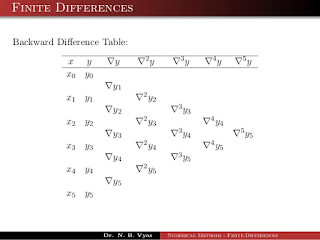## Posts

Showing posts from July, 2019

### What is AI (Artificial Intelligence )? and its characteristicsDefinition : Artificial intelligence  (AI) is the ability of a  computer program  or a  machine  to think and learn. It is also a field of study which tries to make computers "smart". They work on their own without being encoded with commands. Types of AI : REACTIVE MACHINES:  The most basic type of AI is purely reactive ,it does not store any memory of past or predict ( calculate ) future happenings. This type of AI is only applicable to specific applications and the principle is choosing the best decision among several options. examples: Deep Blue, IBM’s chess-playing supercomputer LIMITED MEMORY :  this type 2 kind of AI machines are distinct from reactive machine in such a way that it can make optimum decisions based on the past information ( data ). examples: self driving cars. THEORY OF MIND:  The understanding that people,creatures and objects can have thoughts or emotions which effect their own behavior. This point is the important divide

### Python program for integration using simpsons 1/3 - RuleThe above rule is applicable only when n=6k (multiple of 6 ) ,we just have to find the value of y corresponding to (value) of x ,given by y=f(x). Now for implementation of this formula in the program ,we need to first find the value's of y corresponding to each x : for that we have to convert the entered string by the user to a python expression for calculation of required values and then substituting in simpson's formula. The conversion of string to python expression can be achieved by using lambda function: ep=input("Expression ") f = lambda x: eval(ep) here, the input string is first stored in ep   and then converted to python expression (function) using lambda. SOURCE CODE: # SIMPSONS 1/3 -RULE # SOURCE: QXCODING.COM / JATIN YADAV if __name__ == '__main__': ep=input("Expression ") f = lambda x: eval(ep) a, b = map(int, input("limits ").rstrip().split()) n = int(input("number of strips for accuracy &

### Implementation of NBDF ( Newtons backward difference formula ) in C programThe above table shows the values which are to be calculated by subtracting the corresponding values in the preceding column, and then substituted in the formula given below: Here, p= (x-x0) /h   ; h=common difference; After substituting the differences and calculations we will obtain the highly probable value of the function at the given point. So , now heading for the program: The program contains : 2 recursive functions and one driver function (main()) Recursive functions: int fact(int num){ if(num==0) return 1; return num*fact(num-1); } this functions will return the factorial of the value which is passed to it through actual parameters. and another function float pinc()  : float pinc(float p,float pf){ if(fabs(p-pf)<0.05) return p; else return p*pinc(p-1,pf); } which accepts two arguments p=higher value and pf=lower value , for example: In the term p(p+1)(p+2)(p+3)(p+4)........(p+n-1) : p=p

### Link SQL Database in C/C++ program